Your data analysis solution

# Two-stage least squares regression 2SLS in Excel

2017-10-20

This tutorial will help you set up and interpret a Two-stage least squares regression in Excel with the XLSTAT software.

## Data to run a two-stage least squares regression

The data correspond to the demand-and-supply food example of Kmenta [Kmenta, J. (1971). Elements of Econometrics, 565-582]. This dataset is composed by 5 variables: the food consumption per head (FCH), the ratio of food prices and general price (RFP), the disposable income (DI), the ratio of preceding year price (RPP) and the time in years 1922-1941 (Year). The FCH and the RFP are endogenous variables and the DI, RPP and Year are exogenous.

## Goal of this tutorial

We want to find out how the food consumption varies with the ratio of food prices and general price and the disposable income.

As one of the explanatory variables is exogenous, the DI, RPP and Year variables are used as instrumental and we estimate the parameters by the two-stage least squares method.

## Setting up a two-stage least squares regression

After opening XLSTAT, select the XLSTAT / XLSTAT-MX/ Two-stage least squares command, or click on the corresponding button of the Modeling data toolbar.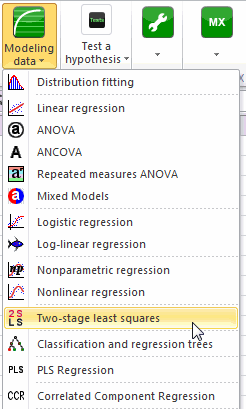Once you've clicked on the button, the dialog box appears.

The data are presented in a table of 20 observations and 5 variables. The FCH is the dependent variable, the RFP and the DI variables are the explanatory variables and the DI, RPP and Year variables are used as instrumental variables. As we selected the column title for the variables, we leave the option Variable labels activated.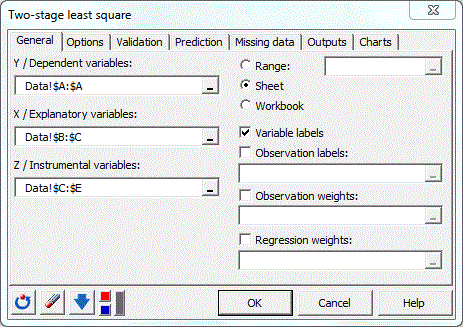On the Options tab, the user can set the tolerance, the level of confidence intervals and the option to include or not the constant in the model. Here we choose to leave the default values.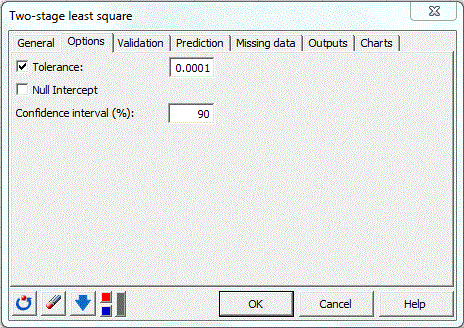The computations begin once you have clicked on OK. The results will then be displayed in a new sheet.

## Interpreting the results

The first results displayed are the statistics for the various variables. Next, a table displays the goodness of fit coefficients of the model. The R² (coefficient of determination) indicates the % of variability of the dependent variable which is explained by the explanatory variables. The closer to 1 the R² is, the better the fit.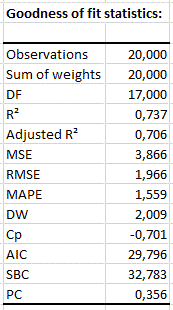It is important to examine the results of the analysis of variance table (see below). The results enable us to determine whether or not the explanatory variables bring significant information (null hypothesis H0) to the model. In other words, it's a way of asking yourself whether it is valid to use the mean to describe the whole population, or whether the information brought by the explanatory variables is of value or not.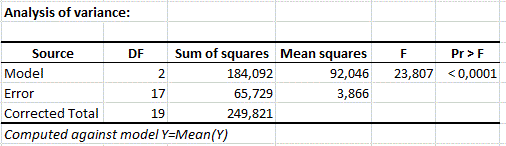The Fisher's F test is used. Given the fact that the probability corresponding to the F value is lower than 0.0001, it means that we would be taking a lower than 0.01% risk in assuming that the null hypothesis (no effect of the two explanatory variable) is wrong. Therefore, we can conclude with confidence that the three variables do bring a significant amount of information.

The following table gives details on the model (parameters, sd, …). This table is helpful when predictions are needed, or when you need to compare the coefficients of the model for a given population with the ones obtained for another population.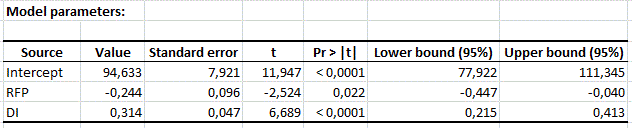Next, table and graphs enable us to take a closer look at each of the standardized residuals. These residuals, should be normally distributed, meaning that 95% of the residuals should be in the interval [-1.96, 1.96]. The histogram of the residuals enables us to quickly visualize the residuals that are out of this interval.Here, none of the values is out of the interval.

### Contact Us

#### Contact our technical support team: support@xlstat.com

https://cdn.desk.com/
false
desk
Loading
seconds ago
a minute ago
minutes ago
an hour ago
hours ago
a day ago
days ago
about
false
Invalid characters found
/customer/portal/articles/autocomplete
9283